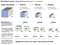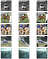# Deep Learning 2: Part 2 Lesson 10

## Review of Last Week [0:16]by Chloe Sultan

# Natural Language Processing [14:10]

## Where we are going :http://forums.fast.ai/t/fun-with-lesson8-rotation-adjustment-things-you-can-do-without-annotated-dataset/14261/1

## IMDb [20:32]

`from fastai.text import *import html`
`BOS = 'xbos'  # beginning-of-sentence tagFLD = 'xfld'  # data field tagPATH=Path('data/aclImdb/')`

## Standardize format [21:27]

`CLAS_PATH=Path('data/imdb_clas/')CLAS_PATH.mkdir(exist_ok=True)LM_PATH=Path('data/imdb_lm/')LM_PATH.mkdir(exist_ok=True)`
`CLASSES = ['neg', 'pos', 'unsup']def get_texts(path):    texts,labels = [],[]    for idx,label in enumerate(CLASSES):        for fname in (path/label).glob('*.*'):            texts.append(fname.open('r').read())            labels.append(idx)    return np.array(texts),np.array(labels)trn_texts,trn_labels = get_texts(PATH/'train')val_texts,val_labels = get_texts(PATH/'test')len(trn_texts),len(val_texts)(75000, 25000)`
`col_names = ['labels','text']`
`np.random.seed(42)trn_idx = np.random.permutation(len(trn_texts))val_idx = np.random.permutation(len(val_texts))`
`trn_texts = trn_texts[trn_idx]val_texts = val_texts[val_idx]trn_labels = trn_labels[trn_idx]val_labels = val_labels[val_idx]`
`df_trn = pd.DataFrame({'text':trn_texts, 'labels':trn_labels},                       columns=col_names)df_val = pd.DataFrame({'text':val_texts, 'labels':val_labels},                      columns=col_names)df_trn[df_trn['labels']!=2].to_csv(CLAS_PATH/'train.csv',                                   header=False, index=False)df_val.to_csv(CLAS_PATH/'test.csv', header=False, index=False)(CLAS_PATH/'classes.txt').open('w')    .writelines(f'{o}\n' for o in CLASSES)(CLAS_PATH/'classes.txt').open().readlines()['neg\n', 'pos\n', 'unsup\n']`
`trn_texts,val_texts = sklearn.model_selection.train_test_split(    np.concatenate([trn_texts,val_texts]), test_size=0.1)len(trn_texts), len(val_texts)(90000, 10000)`
`df_trn = pd.DataFrame({'text':trn_texts, 'labels':                       *len(trn_texts)}, columns=col_names)df_val = pd.DataFrame({'text':val_texts, 'labels':                       *len(val_texts)}, columns=col_names)df_trn.to_csv(LM_PATH/'train.csv', header=False, index=False)df_val.to_csv(LM_PATH/'test.csv', header=False, index=False)`

## Language model tokens [28:03]

`chunksize=24000`
`re1 = re.compile(r'  +')def fixup(x):   x = x.replace('#39;', "'").replace('amp;', '&')        .replace('#146;', "'").replace('nbsp;', ' ')        .replace('#36;', '\$').replace('\\n', "\n")        .replace('quot;', "'").replace('<br />', "\n")        .replace('\\"', '"').replace('<unk>','u_n')        .replace(' @.@ ','.').replace(' @-@ ','-')        .replace('\\', ' \\ ')    return re1.sub(' ', html.unescape(x))def get_texts(df, n_lbls=1):    labels = df.iloc[:,range(n_lbls)].values.astype(np.int64)    texts = f'\n{BOS} {FLD} 1 ' + df[n_lbls].astype(str)    for i in range(n_lbls+1, len(df.columns)):         texts += f' {FLD} {i-n_lbls} ' + df[i].astype(str)    texts = texts.apply(fixup).values.astype(str)    tok = Tokenizer().proc_all_mp(partition_by_cores(texts))    return tok, list(labels)`
`def get_all(df, n_lbls):    tok, labels = [], []    for i, r in enumerate(df):        print(i)        tok_, labels_ = get_texts(r, n_lbls)        tok += tok_;        labels += labels_    return tok, labels`
`df_trn = pd.read_csv(LM_PATH/'train.csv', header=None,                      chunksize=chunksize)df_val = pd.read_csv(LM_PATH/'test.csv', header=None,                      chunksize=chunksize)tok_trn, trn_labels = get_all(df_trn, 1)tok_val, val_labels = get_all(df_val, 1)01230(LM_PATH/'tmp').mkdir(exist_ok=True)`
`' '.join(tok_trn)`
`np.save(LM_PATH/'tmp'/'tok_trn.npy', tok_trn)np.save(LM_PATH/'tmp'/'tok_val.npy', tok_val)tok_trn = np.load(LM_PATH/'tmp'/'tok_trn.npy')tok_val = np.load(LM_PATH/'tmp'/'tok_val.npy')`
`freq = Counter(p for o in tok_trn for p in o)freq.most_common(25)[('the', 1207984), ('.', 991762), (',', 985975), ('and', 587317), ('a', 583569), ('of', 524362), ('to', 484813), ('is', 393574), ('it', 341627), ('in', 337461), ('i', 308563), ('this', 270705), ('that', 261447), ('"', 236753), ("'s", 221112), ('-', 188249), ('was', 180235), ('\n\n', 178679), ('as', 165610), ('with', 159164), ('for', 158981), ('movie', 157676), ('but', 150203), ('film', 144108), ('you', 124114)]`
`max_vocab = 60000min_freq = 2itos = [o for o,c in freq.most_common(max_vocab) if c>min_freq]itos.insert(0, '_pad_')itos.insert(0, '_unk_')`
`stoi = collections.defaultdict(lambda:0,                                {v:k for k,v in enumerate(itos)})len(itos)60002`
`trn_lm = np.array([[stoi[o] for o in p] for p in tok_trn])val_lm = np.array([[stoi[o] for o in p] for p in tok_val])`
`np.save(LM_PATH/'tmp'/'trn_ids.npy', trn_lm)np.save(LM_PATH/'tmp'/'val_ids.npy', val_lm)pickle.dump(itos, open(LM_PATH/'tmp'/'itos.pkl', 'wb'))`
`trn_lm = np.load(LM_PATH/'tmp'/'trn_ids.npy')val_lm = np.load(LM_PATH/'tmp'/'val_ids.npy')itos = pickle.load(open(LM_PATH/'tmp'/'itos.pkl', 'rb'))`
`vs=len(itos)vs,len(trn_lm)(60002, 90000)`

## wikitext103 conversion [46:11]

`# ! wget -nH -r -np -P {PATH} http://files.fast.ai/models/wt103/`
`em_sz,nh,nl = 400,1150,3`
`PRE_PATH = PATH/'models'/'wt103'PRE_LM_PATH = PRE_PATH/'fwd_wt103.h5'`
`wgts = torch.load(PRE_LM_PATH, map_location=lambda storage,                   loc: storage)enc_wgts = to_np(wgts['0.encoder.weight'])row_m = enc_wgts.mean(0)`
`itos2 = pickle.load((PRE_PATH/'itos_wt103.pkl').open('rb'))stoi2 = collections.defaultdict(lambda:-1, {v:k for k,v                                               in enumerate(itos2)})`
`new_w = np.zeros((vs, em_sz), dtype=np.float32)for i,w in enumerate(itos):    r = stoi2[w]    new_w[i] = enc_wgts[r] if r>=0 else row_m`
`wgts['0.encoder.weight'] = T(new_w)wgts['0.encoder_with_dropout.embed.weight'] = T(np.copy(new_w))wgts['1.decoder.weight'] = T(np.copy(new_w))`

## Back to Language Model [1:02:20]

`wd=1e-7bptt=70bs=52opt_fn = partial(optim.Adam, betas=(0.8, 0.99))t = len(np.concatenate(trn_lm))t, t//64(24998320, 390598)`
`trn_dl = LanguageModelLoader(np.concatenate(trn_lm), bs, bptt)val_dl = LanguageModelLoader(np.concatenate(val_lm), bs, bptt)md = LanguageModelData(PATH, 1, vs, trn_dl, val_dl, bs=bs,                        bptt=bptt)`

## Choosing dropout [1:20:36]

`drops = np.array([0.25, 0.1, 0.2, 0.02, 0.15])*0.7learner= md.get_model(opt_fn, em_sz, nh, nl,     dropouti=drops, dropout=drops, wdrop=drops,    dropoute=drops, dropouth=drops)learner.metrics = [accuracy]learner.freeze_to(-1)`

## Measuring accuracy [1:21:45]

`learner.model.load_state_dict(wgts)lr=1e-3lrs = lrlearner.fit(lrs/2, 1, wds=wd, use_clr=(32,2), cycle_len=1)epoch      trn_loss   val_loss   accuracy                         0      4.398856   4.175343   0.28551[4.175343, 0.2855095456305303]learner.save('lm_last_ft')learner.load('lm_last_ft')learner.unfreeze()learner.lr_find(start_lr=lrs/10, end_lr=lrs*10, linear=True)learner.sched.plot()`
`learner.fit(lrs, 1, wds=wd, use_clr=(20,10), cycle_len=15)epoch      trn_loss   val_loss   accuracy                         0      4.332359   4.120674   0.289563      1      4.247177   4.067932   0.294281                         2      4.175848   4.027153   0.298062                         3      4.140306   4.001291   0.300798                         4      4.112395   3.98392    0.302663                         5      4.078948   3.971053   0.304059                         6      4.06956    3.958152   0.305356                         7      4.025542   3.951509   0.306309                         8      4.019778   3.94065    0.30756                          9      4.027846   3.931385   0.308232                         10     3.98106    3.928427   0.309011                         11     3.97106    3.920667   0.30989                          12     3.941096   3.917029   0.310515                         13     3.924818   3.91302    0.311015                         14     3.923296   3.908476   0.311586[3.9084756, 0.3115861900150776]`
`learner.save('lm1')learner.save_encoder('lm1_enc')`

## `save_encoder` [1:31:55]

`learner.sched.plot_loss()`

## Classifier tokens [1:32:31]

`df_trn = pd.read_csv(CLAS_PATH/'train.csv', header=None,                      chunksize=chunksize)df_val = pd.read_csv(CLAS_PATH/'test.csv', header=None,                      chunksize=chunksize)tok_trn, trn_labels = get_all(df_trn, 1)tok_val, val_labels = get_all(df_val, 1)0101(CLAS_PATH/'tmp').mkdir(exist_ok=True)np.save(CLAS_PATH/'tmp'/'tok_trn.npy', tok_trn)np.save(CLAS_PATH/'tmp'/'tok_val.npy', tok_val)np.save(CLAS_PATH/'tmp'/'trn_labels.npy', trn_labels)np.save(CLAS_PATH/'tmp'/'val_labels.npy', val_labels)tok_trn = np.load(CLAS_PATH/'tmp'/'tok_trn.npy')tok_val = np.load(CLAS_PATH/'tmp'/'tok_val.npy')`
`itos = pickle.load((LM_PATH/'tmp'/'itos.pkl').open('rb'))stoi = collections.defaultdict(lambda:0, {v:k for k,v in                                           enumerate(itos)})len(itos)60002trn_clas = np.array([[stoi[o] for o in p] for p in tok_trn])val_clas = np.array([[stoi[o] for o in p] for p in tok_val])np.save(CLAS_PATH/'tmp'/'trn_ids.npy', trn_clas)np.save(CLAS_PATH/'tmp'/'val_ids.npy', val_clas)`

## Classifier

`trn_clas = np.load(CLAS_PATH/'tmp'/'trn_ids.npy')val_clas = np.load(CLAS_PATH/'tmp'/'val_ids.npy')trn_labels = np.squeeze(np.load(CLAS_PATH/'tmp'/'trn_labels.npy'))val_labels = np.squeeze(np.load(CLAS_PATH/'tmp'/'val_labels.npy'))`
`bptt,em_sz,nh,nl = 70,400,1150,3vs = len(itos)opt_fn = partial(optim.Adam, betas=(0.8, 0.99))bs = 48min_lbl = trn_labels.min()trn_labels -= min_lblval_labels -= min_lblc=int(trn_labels.max())+1`

## TextDataset [1:33:37]

`trn_ds = TextDataset(trn_clas, trn_labels)val_ds = TextDataset(val_clas, val_labels)`

## Turning it to a DataLoader [1:36:27]

`trn_samp = SortishSampler(trn_clas, key=lambda x: len(trn_clas[x]),                           bs=bs//2)val_samp = SortSampler(val_clas, key=lambda x: len(val_clas[x]))trn_dl = DataLoader(trn_ds, bs//2, transpose=True, num_workers=1,                    pad_idx=1, sampler=trn_samp)val_dl = DataLoader(val_ds, bs, transpose=True, num_workers=1,                     pad_idx=1, sampler=val_samp)md = ModelData(PATH, trn_dl, val_dl)`
` # part 1dps = np.array([0.4, 0.5, 0.05, 0.3, 0.1])dps = np.array([0.4,0.5,0.05,0.3,0.4])*0.5m = get_rnn_classifer(bptt, 20*70, c, vs, emb_sz=em_sz, n_hid=nh,                       n_layers=nl, pad_token=1,                      layers=[em_sz*3, 50, c], drops=[dps, 0.1],                      dropouti=dps, wdrop=dps,                              dropoute=dps, dropouth=dps)opt_fn = partial(optim.Adam, betas=(0.7, 0.99))`
`learn = RNN_Learner(md, TextModel(to_gpu(m)), opt_fn=opt_fn)learn.reg_fn = partial(seq2seq_reg, alpha=2, beta=1)learn.clip=25.learn.metrics = [accuracy]`
`lr=3e-3lrm = 2.6lrs = np.array([lr/(lrm**4), lr/(lrm**3), lr/(lrm**2), lr/lrm, lr])lrs=np.array([1e-4,1e-4,1e-4,1e-3,1e-2])`
`wd = 1e-7wd = 0learn.load_encoder('lm2_enc')`
`learn.freeze_to(-1)learn.lr_find(lrs/1000)learn.sched.plot()learn.fit(lrs, 1, wds=wd, cycle_len=1, use_clr=(8,3))epoch      trn_loss   val_loss   accuracy                          0      0.365457   0.185553   0.928719[0.18555279, 0.9287188090884525]learn.save('clas_0')learn.load('clas_0')`
`learn.freeze_to(-2)learn.fit(lrs, 1, wds=wd, cycle_len=1, use_clr=(8,3))epoch      trn_loss   val_loss   accuracy                          0      0.340473   0.17319    0.933125[0.17319041, 0.9331253991245995]learn.save('clas_1')learn.load('clas_1')learn.unfreeze()learn.fit(lrs, 1, wds=wd, cycle_len=14, use_clr=(32,10))epoch      trn_loss   val_loss   accuracy                          0      0.337347   0.186812   0.930782      1      0.284065   0.318038   0.932062                          2      0.246721   0.156018   0.941747                          3      0.252745   0.157223   0.944106                          4      0.24023    0.159444   0.945393                          5      0.210046   0.202856   0.942858                          6      0.212139   0.149009   0.943746                          7      0.21163    0.186739   0.946553                          8      0.186233   0.1508     0.945218                          9      0.176225   0.150472   0.947985                          10     0.198024   0.146215   0.948345                          11     0.20324    0.189206   0.948145                          12     0.165159   0.151402   0.947745                          13     0.165997   0.146615   0.947905[0.14661488, 0.9479046703071374]learn.sched.plot_loss()learn.save('clas_2')`

# Tricks to run ablation studies [1:58:32]

## Trick #2: Google Fire [2:01:27]

`if __name__ == '__main__': fire.Fire(train_clas)`

## Trick #5: SentencePiece [2:05:06]

Welcome to a place where words matter. On Medium, smart voices and original ideas take center stage - with no ads in sight. Watch
Follow all the topics you care about, and we’ll deliver the best stories for you to your homepage and inbox. Explore
Get unlimited access to the best stories on Medium — and support writers while you’re at it. Just \$5/month. Upgrade